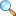# Chapter 09: First Order Differential Equations

Notes of the book Mathematical Method written by S.M. Yusuf, A. Majeed and M. Amin, published by Ilmi Kitab Khana, Lahore - PAKISTAN.

• D.E and their classification
• Formation of differential equation
• Initial and boundary conditions
• Separable equations
• Homogeneous equations
• D.E. Reducible to homogeneous form
• Exercise 9.2 by Umer Asghar |View online | Download PDF
• bsc/notes_of_mathematical_method/ch09_first_order_differential_equations